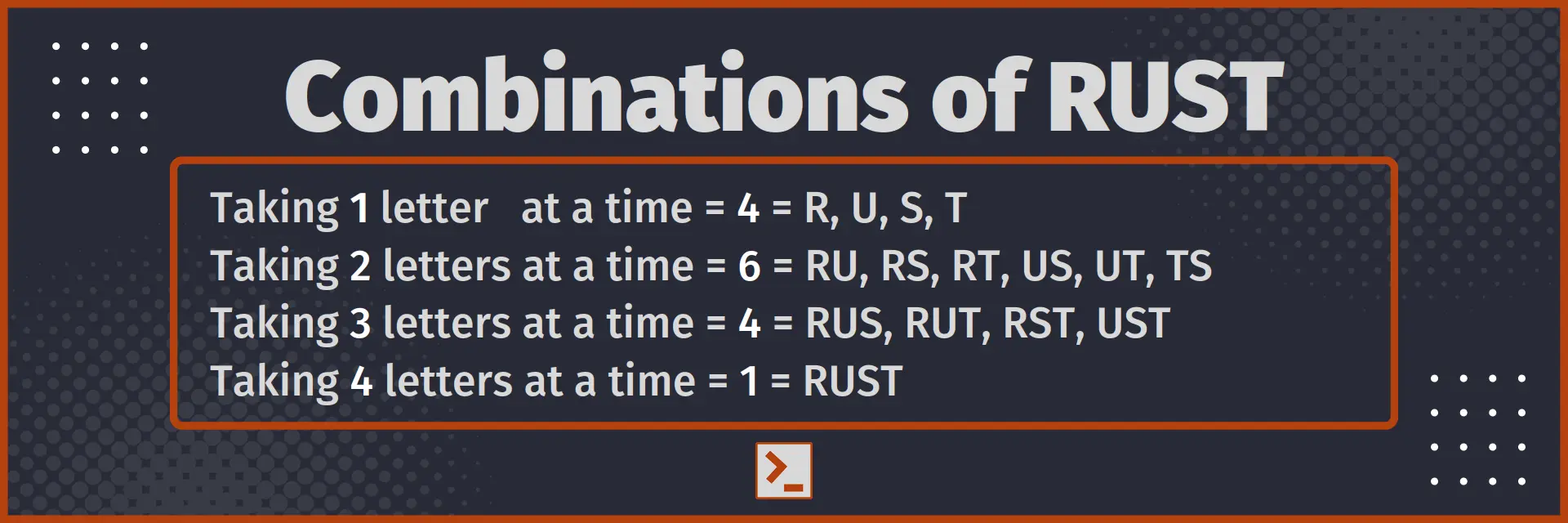# Combination

and program to find the Combination in Rust

## What is Combination

Combination is the number of ways in which we can choose some objects from the given set of objects. In combination, the order in which things are arranged do not matter, unlike in permutation.

For example : The Combinations of word RUST of length 3 are 4 => RUS, RUT, RST, UST.

In this article, we will use standard reference : the number of total objects in the set are denoted by n and the number of items chosen at a time are denoted by r.

So, total number of ways of choosing r items from n items are represented as nCr. It will also be written as C(n, r)## Combination formulae

Here are some frequently used formulae for Combinations.

1. nCr = n ! / ( (n-r)! × r! )
2. nC0 + nC1 + nC2 ..... + nCn = 2n

## Program to find Combination

Now, let us write a program in Rust Language to find the number of permutations when we are given n distinct objects, and we can arrange r at a time.

`fn combination(n: usize, r: usize) -> usize{    // nCr = n! / (r! * (n-r)!)    return factorial(n)/(factorial(r) * factorial(n-r));}`

With driver code

```fn combination(n: usize, r: usize) -> usize{    // nCr = n! / (r! * (n-r)!)    return factorial(n)/(factorial(r) * factorial(n-r));}
// Driver code
use std::io::stdin;fn take_int() -> usize {    let mut input = String::new();    stdin().read_line(&mut input).unwrap();    return input.trim().parse().unwrap();}fn factorial(number : usize) -> usize{    let mut factorial : usize = 1;    for i in 1..(number+1) { factorial*=i; }    return factorial;}
pub fn main() {    let n = take_int();    let r = take_int();    println!("{}", combination(n, r));}```

Input

6
4

Output

15

Time Complexity : O( n )
Space Complexity : O( 1 )

## Conclusion

Combination is the number of ways in which we can choose some objects from the given set of objects. In this article, we saw the frequently used formulae of combinations for given n and r and also made a program to find number of combinations in Rust.

Here is the function for easy access

```fn combination(n: usize, r: usize) -> usize{    return factorial(n)/(factorial(r) * factorial(n-r));}
fn factorial(number : usize) -> usize{    let mut factorial : usize = 1;    for i in 1..(number+1) { factorial*=i; }    return factorial;}```

Thank You